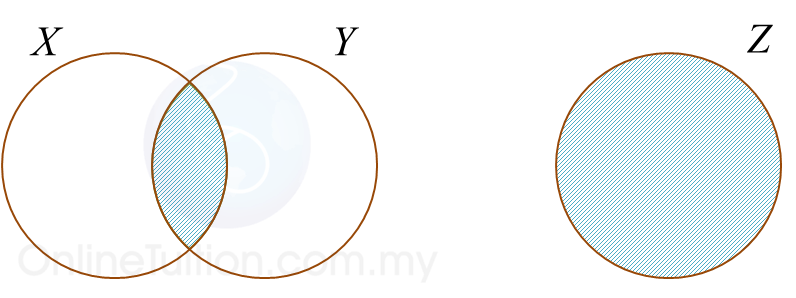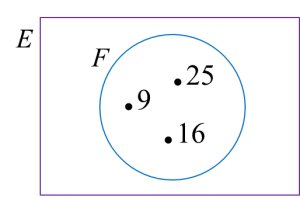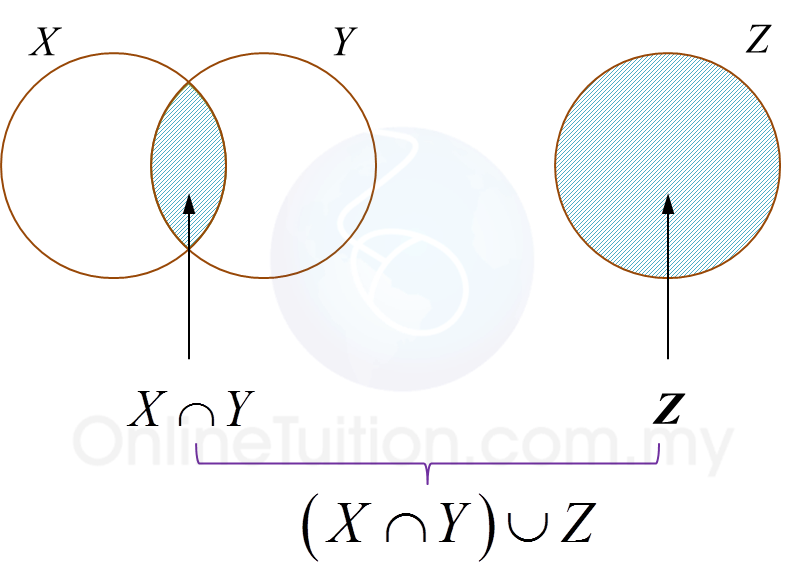# SPM Mathematics 2018, Paper 2 (Questions 1 & 2)

Question 1 (3 marks):
(a) It is given that set E = {perfect square numbers} and set F = {9, 16, 25}.
Complete the Venn diagram in the answer space to show the relationship between set E and set F.(b)
The Venn diagram in Diagram 1 shows the sets X, set Y and set Z.
The universal set, ξ = X U Y U Z.Diagram 1

State the relationship represented by the shaded region between sets X, set Y and set Z.

Solution:

(a)(b)Hence, relationship represented by the shaded region between sets X, set Y and set are (X ∩ Y) ∪ Z.

Question 2 (3 marks):
Diagram 2 shows a cuboid PQRSTUVW on a horizontal plane. Point N is the midpoint of QR.Diagram 2

(a)
Name the angle between the plane TWN and the plane PQRS.
(b) Calculate the angle between the plane TWN and the plane PQRS.

Solution:
(a)
Angle between the plane TWN and the plane PQRS
= ∠ WNR

(b)$\begin{array}{l}\mathrm{tan}\angle WNR=\frac{4}{3}\\ \angle WNR={53.13}^{o}\end{array}$